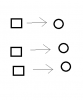# GMS 2Passing instance ID to other instance

#### sparklemotion

##### Member
Hi all

I am attempting to have an instance of obj_square to follow obj_circle. The issue is that I have several instances of obj_square present, and several instances of obj_circle
present. I have attempted to draw the setup in the image attached.

What I need is for one instance of obj_square to move towards one instance of obj_circle. So, they need to be paired up - I think - maybe by having one instance of
obj_circle pass its ID to one instance of obj_square.

Any advice how I could do this?

Thanks a lot!#### Skere

##### Member
Something like this?
GML:
``````var circle = instance_create_layer(0, 0, "Instances", obj_circle);
var square = instance_create_layer(0, 0, "Instances", obj_square);
square.circleId = circle;``````
Then you can use circleId in the step event of obj_square to make it follow the circle's position.

#### woods

##### Member
something like this?

var nearest_circle = instance_nearest(x, y, obj_circle);
move_towards_point(nearest_circle.x, nearest_circle.y, move_speed);

#### FrostyCat

##### Member
See: What's the Difference: Objects and Instances (Finding the right instance)
Created or copied instance. instance_create() (GMS 1.x and legacy) / instance_create_layer() and instance_create_depth() (GMS 2.x) and instance_copy() return the ID of the created or copied instance. NEVER use instance_nearest() to establish this relationship --- something else could be closer by.

Subsidiary instance(s). Same as created or copied instance. If the subsidiary instance needs to reference its creator, the creator should assign its instance ID to an instance variable in the subsidiary instance. Conversely, if the creator needs to reference its subsidiary (or subsidiaries), it must store the return value of instance_create() (GMS 1.x and legacy) / instance_create_layer() or instance_create_depth() (GMS 2.x) immediately. NEVER use instance_nearest() to establish or maintain this relationship --- being the closest does NOT imply being the most relevant, especially in close quarters.
Circle Create:
GML:
``````mySquare = instance_create_layer(x, y, layer, obj_square);
mySquare.myCircle = id;``````
Square Step:
GML:
``````var spd = 4;
if (point_distance(x, y, myCircle.x, myCircle.y) <= spd) {
x = myCircle.x;
y = myCircle.y;
speed = 0;
} else {
move_towards_point(myCircle.x, myCircle.y, spd);
}``````

#### sparklemotion

##### Member
Thanks for your help. I am still trying to figure this out, maybe I should explain the direct application of the instances better.

GML:
``mySquare.myCircle = id;``
What does it do?

The setup I need is this: Let's say I press a button, which creates one "obj_square" instance. If I press the button five times, it creates five "obj_square" instances. Now, when I press another button I choose the first "obj_square" instance, pressing the same button again cycles to the next "obj_square" instance in the queue. When a "obj_square" instance is 'selected', an "obj_circle" instance is created at the same x- and y-position as the "obj_square" instance. You are then able to move the "obj_circle" instance to a place in the room, and when you press the 'cycle' button, which cycles to the next "obj_square" instance, the de-selected instance will move to where the "obj_circle" instance was placed.

I have most of this working right now, but I do have some problems with getting the last code down. Would it be better if I attached the GMS2-file insetad? It is not a lot of code to "skim through"Thanks again!

#### woods

##### Member
from what i can gather from frosty's bit of code, basically..
when mySquare is created, the variable myCircle contains the ID of that particular obj_square.. which is used to move to that circle.

effectively keeping that circle and that square "linked"

•sparklemotion

#### FrostyCat

##### Member

GML:
``mySquare.myCircle = id;``
What does it do?
You would know exactly what it does if you read the Manual entries for `instance_create_layer()` and `id`.
GML:
``````// Create an instance of the square and remember its instance ID in mySquare
mySquare = instance_create_layer(x, y, layer, obj_square);
// Set a myCircle variable in that new instance remembering the current instance's ID
mySquare.myCircle = id;``````
The setup I need is this: Let's say I press a button, which creates one "obj_square" instance. If I press the button five times, it creates five "obj_square" instances. Now, when I press another button I choose the first "obj_square" instance, pressing the same button again cycles to the next "obj_square" instance in the queue. When a "obj_square" instance is 'selected', an "obj_circle" instance is created at the same x- and y-position as the "obj_square" instance. You are then able to move the "obj_circle" instance to a place in the room, and when you press the 'cycle' button, which cycles to the next "obj_square" instance, the de-selected instance will move to where the "obj_circle" instance was placed.
Then just add the new square instance IDs to a list instead of a single variable.

Really, none of this is difficult if you would just accept that object IDs are not the only things that belongs on the left side of a dot.

#### sparklemotion

##### Member
Hi guys. Sorry for the late reply.

Well, I managed to figure it out - it works now. I know I have some problems with coding once in a while, but I still think I am progressing. It's a fun hobby!

Thanks!

•woods